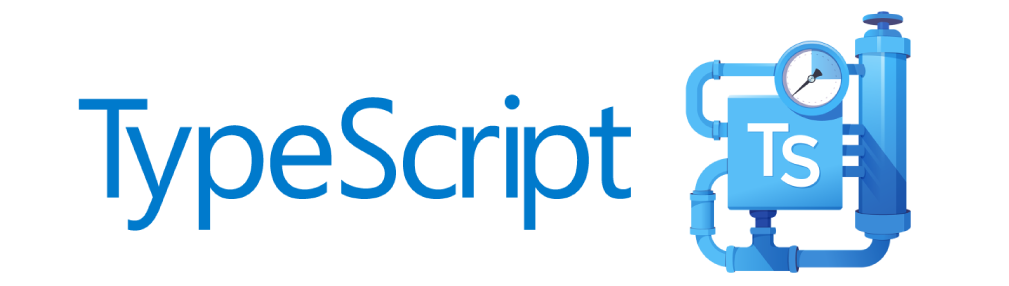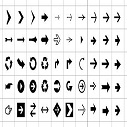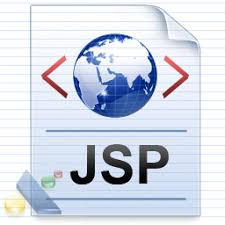# PL/SQL MAP Member Functions

The MAP member functions are used for performing comparisons between a single attribute of an object instance to a single attribute of another object instance.

The MAP functions do not accept any formal parameters and must return a scalar data type, either CHAR, VARCHAR2, NUMBER or DATE, which can be easily compared by the system. The MAP member functions are used for validating object instances with a single attribute.

Note -The MAP functions cannot be implemented on Static functions, but Member functions only.

```CREATE OR REPLACE TYPE obj_map AS OBJECT
(
obj_var1 NUMBER,
MEMBER PROCEDURE print,
MAP MEMBER FUNCTION func_map RETURN NUMBER
);

CREATE OR REPLACE TYPE BODY obj_map
IS
MEMBER PROCEDURE print
IS
BEGIN
dbms_output.put_line(self.obj_var1);
END;
MAP MEMBER FUNCTION func_map RETURN NUMBER
IS
BEGIN
RETURN obj_var1;
END;
END;```

In the above snippet, an object is created with an attribute of the number data type, a member procedure for printing the attribute value of the current instance and an MAP member function returning the attribute of the current instance.

The attribute value for multiple instances can be compared with the help of the below anonymous block which has two instances of the object OBJ_MAP, which accepts bind values as their initialization parameter. In the execution section, the attribute values of the two instances of the objects are printed using the print procedure from the object.

The IF condition merely checks for the equality between the two object instances, which internally calls the MAP member function and compares the result of the two instance's MAP member function's return value, which is the attribute value of the two instances ideally. The result of the comparison between the two object instance's attributes is printed appropriately using the PUT_LINE procedure.

```DECLARE
l_obj_map1 obj_map:=obj_map(:l_obj_map1);
l_obj_map2 obj_map:=obj_map(:l_obj_map2);
BEGIN
l_obj_map1.print;
l_obj_map2.print;
IF l_obj_map1=l_obj_map2 THEN
dbms_output.put_line('The two intances are equal');
ELSE
dbms_output.put_line('The two intances are not equal');
END IF;
END;```

### Result:

1. When l_obj_map1= 30 and l_obj_map2= 50 then,

```30
50
The two intances are not equal```

2. When l_obj_map1= 50 and l_obj_map2= 50 then,

```50
50
The two intances are equal```Admin on Oct 09, 2019 at 12:10 am

If you like dEexams.com and would like to contribute, you can write your article here or mail your article to admin@deexams.com . See your article appearing on the dEexams.com main page and help others to learn.

#### Post Comment

##### WEB TECHNOLOGY##### TypeScript Programmning Language##### New HTML5 APIs##### HTML Arrow -> Range: Decimal 8592-8703. Hex 2190-21FF.##### How To Override One CSS Class With Another×